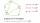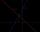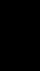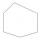# Algebra - math word problems

#### Number of problems found: 2801

• Sum of inner anglesProve that the sum of all inner angles of any convex n-angle equals (n-2) . 180 degrees.
• DigitsShow that if x, y, z are 3 consecutive nonzero digits, zyx-xyz = 198, where zyx and xyz are three-digit numbers created from x, y, z.
• Geometric progression 48,4√2,4,2√2
• AlgebraX+y=5, find xy (find the product of x and y if x+y = 5)
• IntersectionsFind the intersections of the function plot with coordinate axes: f (x): y = x + 3/5
• Utopia IslandA probability of disease A on the island of Utopia is 40%. A probability of occurrence among the men of this island, which make up 60% of all the population (the rest are women), is 50%. What is the probability of occurrence of A disease among women on Ut
• Regular prismThe regular four-sided prism has a base of 25 cm2 and a surface of 210 cm2. Find its volume.
• 40% volume40% volume with 104 uph (units per labor hour) 8 people working. What is the volume?
• Cube edgesThe sum of the lengths of the cube edges is 42 cm. Calculate the surface of the cube.The cone-shaped lampshade has a diameter of 30 cm and a height of 10 cm. How many cm2 of material will we need when we 10% is waste?
• EllipseEllipse is expressed by equation 9x2 + 25y2 - 54x - 100y - 44 = 0. Find the length of primary and secondary axes, eccentricity, and coordinates of the center of the ellipse.
• In a 2In a thirteen sided polygon, the sum of five angles is 1274°, four of the eight angles remaining are equal and the other four are 18° less than each of the equal angles. Find the angles. .
• Six-sided polygonIn a six-sided polygon. The first two angles are equal, the third angle is twice (the equal angles), two other angles are trice the equal angle, while the last angle is a right angle. Find the value of each angle.
• DitchDitch profile is an isosceles trapezoid with bases of length 80m and 60m. The slope of the side wall of the ditch is 80°. Calculate the ditch depth.
• Area of iso-trapFind the area of an isosceles trapezoid if the lengths of its bases are 16 cm and 30 cm, and the diagonals are perpendicular to each other.
• Simplify 2Simplify expression: 5ab-7+3ba-9
• Intercept with axisF(x)=log(x+4)-2, what is the x intercept
• Right triangle eq2Find the lengths of the sides and the angles in the right triangle. Given area S = 210 and perimeter o = 70.
• Ratio of edgesThe dimensions of the cuboid are in a ratio 3: 1: 2. The body diagonal has a length of 28 cm. Find the volume of a cuboid.
• A squareA square with length of diagonals 12cm give: a) Calculate the area of a square b) rhombus with the same area as the square, has one diagonal with length of 16 cm. Calculate the length of the other diagonal.

Do you have an interesting mathematical word problem that you can't solve it? Submit a math problem, and we can try to solve it.

We will send a solution to your e-mail address. Solved examples are also published here. Please enter the e-mail correctly and check whether you don't have a full mailbox.

Please do not submit problems from current active competitions such as Mathematical Olympiad, correspondence seminars etc...